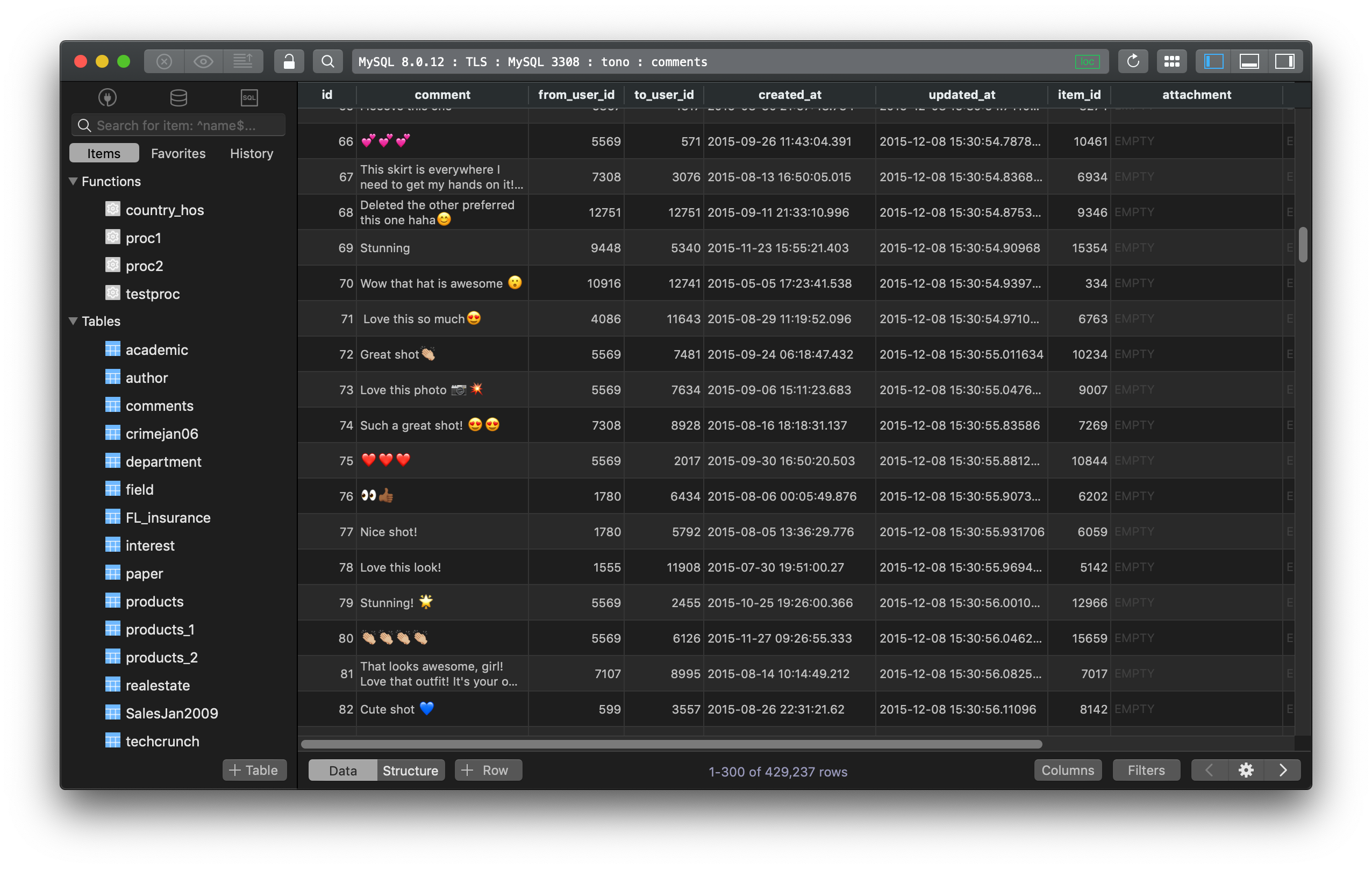You have a table students with `id`, `score1`, and `score2` like this, where `id` is the primary key:

id score1 score2
1 10 9
2 8 3
3 10 6
4 4 8

And here is the new table of data that you want to update to:

id score1 score2
1 5 8
2 10 8
3 8 3
4 10 7

There are a couple of ways to do it.

1. You can either write multiple UPDATE queries like this and run them all at once:

``````UPDATE students SET score1 = 5, score2 = 8 WHERE id = 1;
UPDATE students SET score1 = 10, score2 = 8 WHERE id = 2;
UPDATE students SET score1 = 8, score2 = 3 WHERE id = 3;
UPDATE students SET score1 = 10, score2 = 7 WHERE id = 4;
``````

2. Or you can UPDATE with JOIN statement:

``````UPDATE students s
JOIN (
SELECT 1 as id, 5 as new_score1, 8 as new_score2
UNION ALL
SELECT 2, 10, 8
UNION ALL
SELECT 3, 8, 3
UNION ALL
SELECT 4, 10, 7
) vals ON s.id = vals.id
SET score1 = new_score1, score2 = new_score2;
``````

3. Or you can use `INSERT ... ON DUPLICATE KEY UPDATE`

``````INSERT INTO students
(id, score1, score2)
VALUES
(1, 5, 8),
(2, 10, 8),
(3, 8, 3),
(4, 10, 7)
ON DUPLICATE KEY UPDATE
score1 = VALUES(score1),
score2 = VALUES(score2);
``````

Need a good GUI Tool for MySQL? TablePlus is a modern, native tool with an elegant UI that allows you to simultaneously manage multiple databases such as MySQL, PostgreSQL, SQLite, Microsoft SQL Server and more.